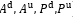# Xlog(1+y/x) in gp mode

Hi everyone,
I have the term xlog(1+ cy/x) in my optimization problem where x and y are variables and c is constant. when I formulate it as -rel_entr(x, x+ c*y) in cvx, it works well, but when i run cvx in gp mode, I get the following error

Disciplined convex programming error:
Illegal operation: rel_entr( {log-affine}, {log-convex} ).

Could you help me to handle this error?

I think you are out of luck with that expression in gp mode.

Can you enter the whole problem without using gp mode? If not, have you proven that the problem is convex, or transformable into a convex problem, in which case that may guide you how to formulate for CVX.

The main problem is as follows

The objective function of the problem is concave.The constraints c7 and c8 are convex and other constraints are all linear. As a result I think the problem is convex.

From my guess as to what the optimization variables are (lower case letters for which corresponding upper case is listed as variable?), it appears that the objective function has product term(s) inside the term multiplying \eta. Please show your proof that is concave.

You can’t mix and match rules of gp mode and non-gp mode in one problem, because that is not necessarily convex or convertible thereto.

I rewrite the objective function as follows

the optimization variables are shown in blue color and all other parameters are constant . I think the first two sigma expressions are concave and the last two expressions are linear , so the objective function is concave

If that is the case, then use `'rel_entr" for the perspective functions, and enter the problem in an otherwise straightforward manner not using gp mode. Does some other difficulty arise in attempting to do so?

Actually, in my main problem the parameter x is a variable too, but for make the problem solvable I decompose it into two sub-problems where at first step, the variablesare optimized by assuming fixed X, and then the optimal X is derived by fixing the obtained. I repeat this procedure until steady state is achieved.
I wonder if I could use the gp mode, I could solve the main problem with all variables at once! so can I use the gp mode for solving this problem?

As far as I can figure out what your problem is, which is not very well, I don’t think you can use gp mode.

As to whether your alternating optimization will converge to anything, and if it does, whether that will be a local optimum, let alone a global optimum …??? Of course, that may depend on your starting values for variables being initially fixed. You may be better off using a non-convex nonlinear optimization solver.

You are right, the proposed method could converge to local optimum and not a global optimum. I would try a non-convex solver.